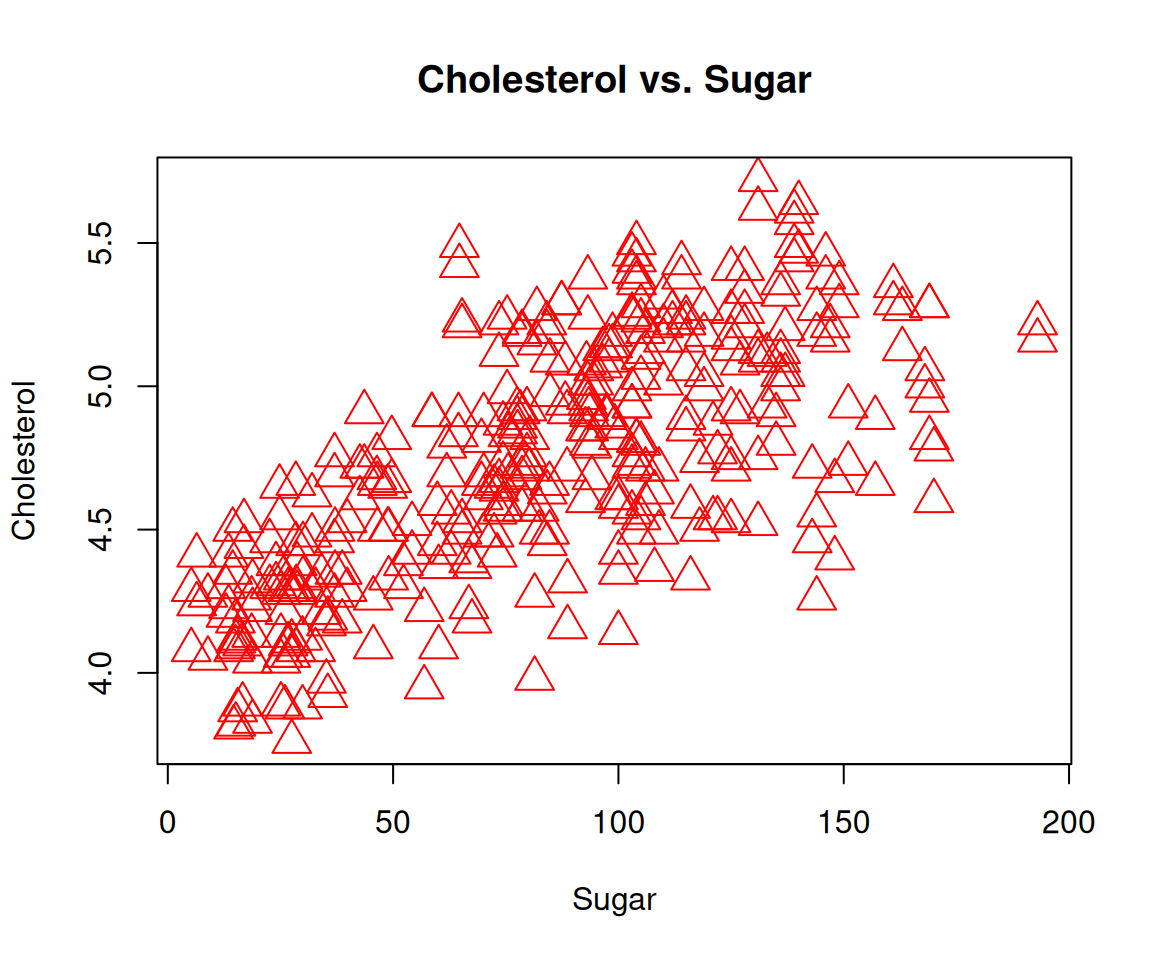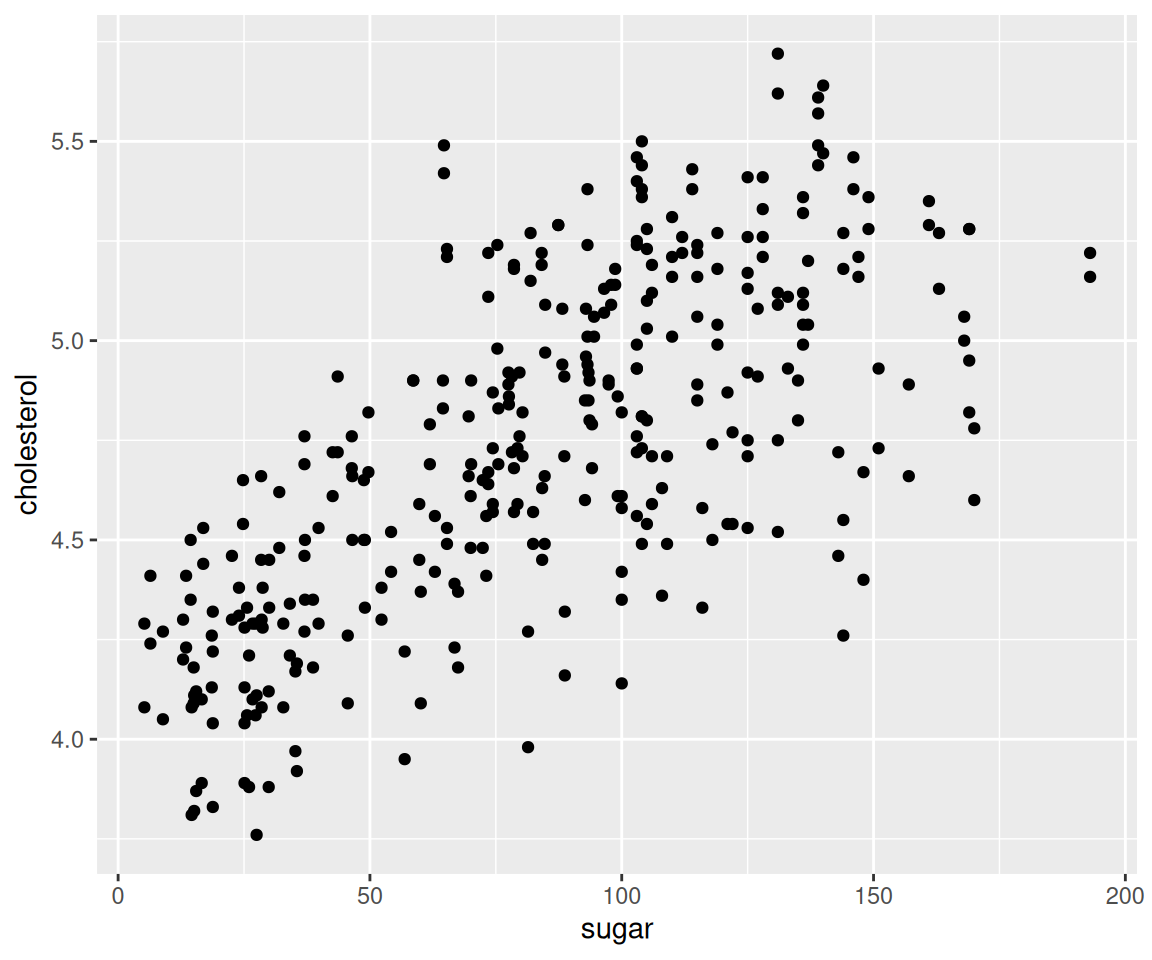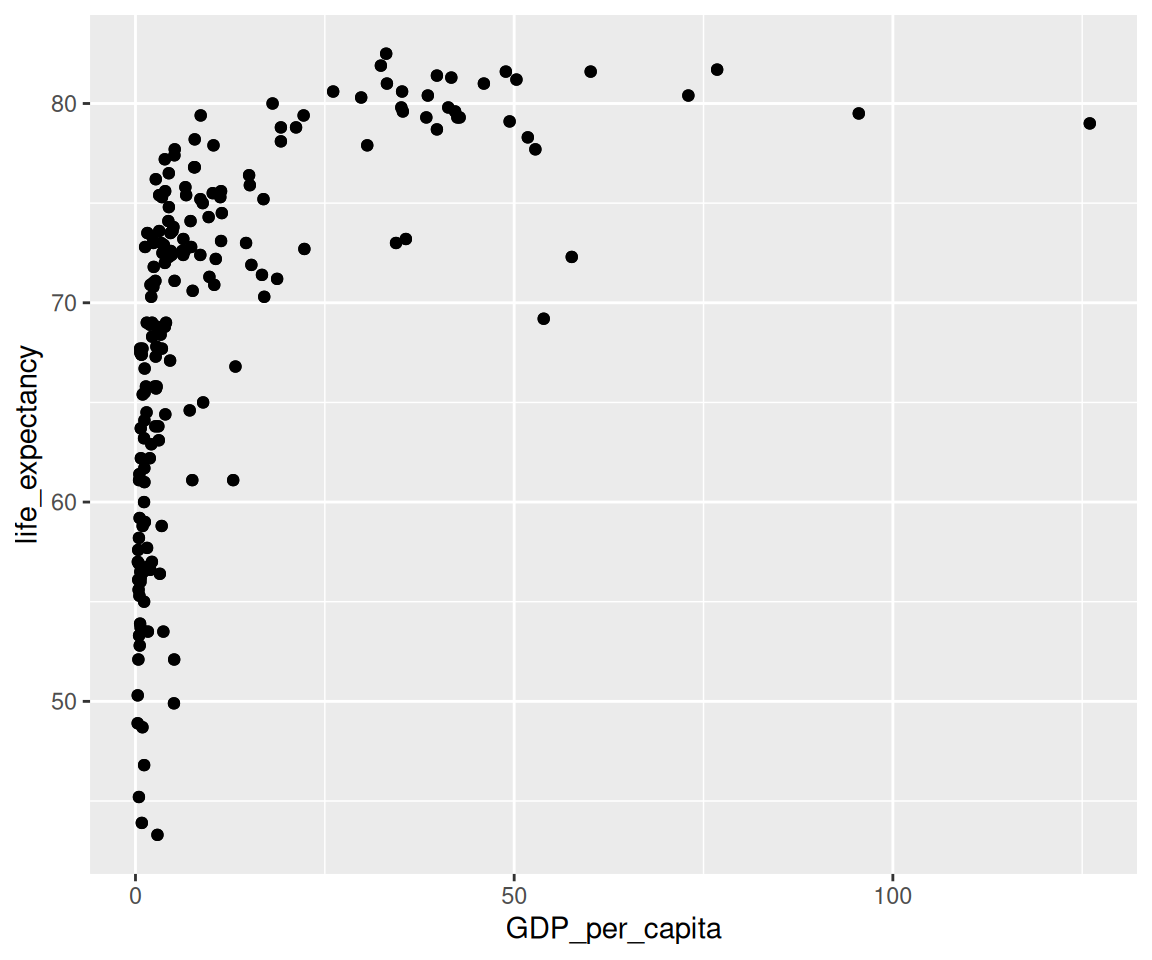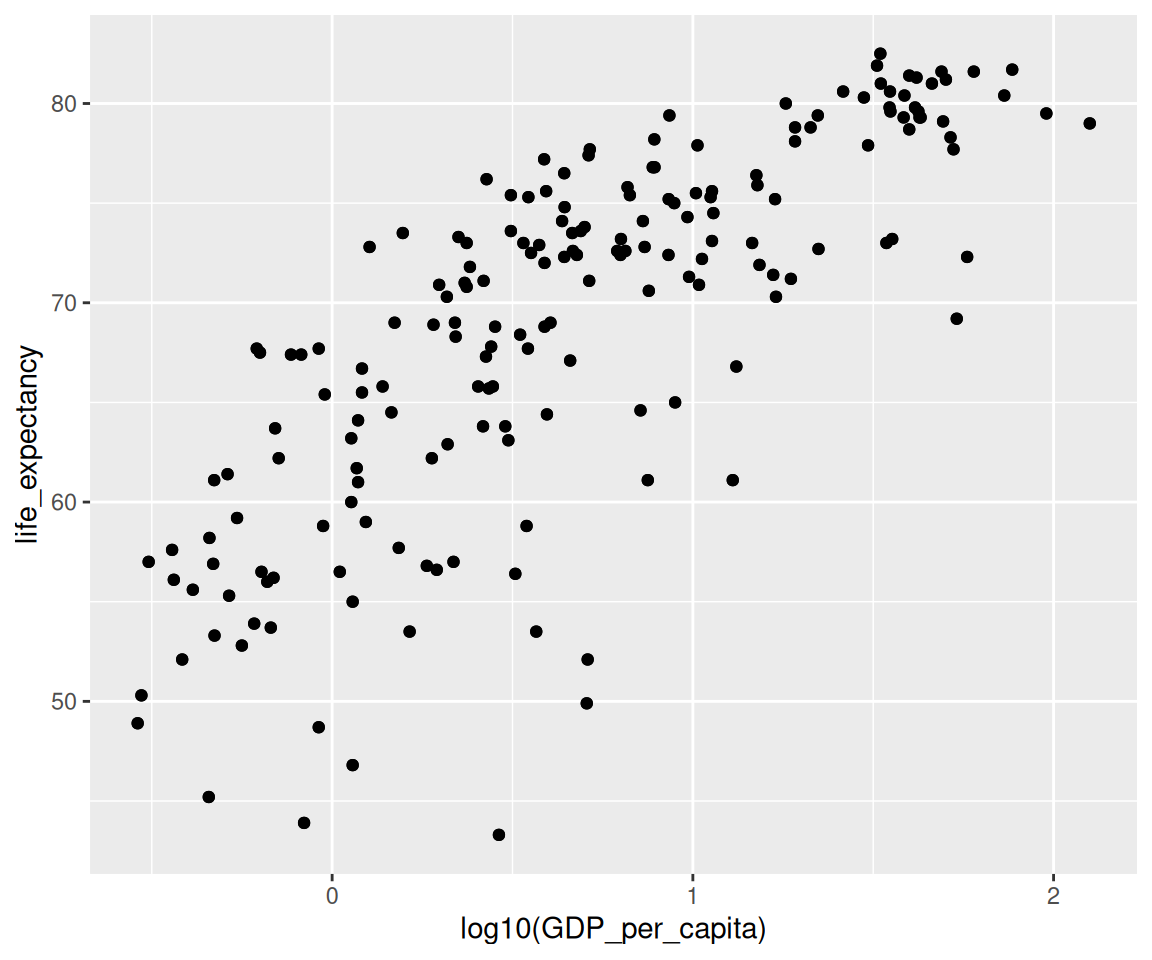## 4.13 Density Plots

In Section 4.12, we have learned to use geom_histogram() as a way to visualize the distribution of a continuous variable. In addition, we can also use it to generate a piece-wise constant estimate of the probability density function. Today, we will introduce another visualization method for continuous data, namely the density plots. First, let’s review the geom_histogram() for estimating the density function.

library(ggplot2)
library(r02pro)
ggplot(data = sahp) +
geom_histogram(aes(x = sale_price, y = ..density..))
#> stat_bin() using bins = 30. Pick better value with binwidth.There are 30 bins by default. You may notice that this density estimate is not smooth, sometimes we may prefer a smoothed estimate. Then, we can use the geom_density() function to achieve this.

ggplot(data = sahp) +
geom_density(aes(x = sale_price))This plot shows the so-called “kernel density estimate,” a popular way to estimate the probability density function from sample. The density estimate can be viewed as a smoothed version of the histogram. We can combine the two plots together using global mapping.

ggplot(data = sahp, aes(x = sale_price)) +
geom_histogram(aes(y = ..density..)) +
geom_density(color = "red", size = 2)
#> stat_bin() using bins = 30. Pick better value with binwidth.Here, we added some global aesthetics in geom_density() to make the density plot red and the line width by setting size = 2. It is clear that the density plot is a useful alternative to the histogram for visualizing continuous data.

### 4.13.1 Aesthetics in Density Plots

Now, let’s introduce some commonly used aesthetics for density plots.

a. Color

ggplot(data = remove_missing(sahp, vars = "oa_qual")) +
geom_density(aes(x = sale_price, color = oa_qual > 5))Here, we divide the data into two groups according to the value of oa_qual, then generate separate density estimates with different colors for oa_qual > 5 and oa_qual <= 5. The blue curve represents the density estimates for larger values of oa_qual while the red curve corresponds to that of the houses with smaller values.

b. Fill

Another way to generate different density estimates is to use the fill aesthetic. Let’s see the following example.

ggplot(data = remove_missing(sahp, vars = "oa_qual")) +
geom_density(aes(x = sale_price, fill = oa_qual > 5))The fill aesthetic also divides the data into groups according to oa_qual, then generate separate density estimates. The difference between fill and color aesthetics is that fill generates shaded areas below each density curve with different colors while color generates density curves with different colors. As we can see from the plot, there is a substantial overlap of the shaded areas. To fix this issue, we can change the transparency of the shades by adjusting the value of the alpha aesthetic.

ggplot(data = remove_missing(sahp, vars = "oa_qual")) +
geom_density(aes(x = sale_price, fill = oa_qual > 5),
alpha = 0.5)We can now see both shaded areas in a clearly way.

c. Linetype

We can also use different linetypes for different curves.

ggplot(data = remove_missing(sahp, vars = "oa_qual")) +
geom_density(aes(x = sale_price, linetype = oa_qual > 5))d. Global aesthetics

As usual, we can also set global aesthetics for geom_density() and combine it with the mapped aesthetics.

ggplot(data = remove_missing(sahp, vars = "oa_qual")) +
geom_density(aes(x = sale_price, linetype = oa_qual > 5),
size = 1,
color = "red")Here, the size controls the width of the density curve.

### 4.13.2 Exercises

Use the sahp data set to answer the following questions.

1. Create density plot on the living area (liv_area) with dashed lines and different colors for different values of kit_qual. What conclusions can you draw from the plot?

2. Try to create density plot for kit_qual. Do you think this plot is informative? If not, create a plot that captures the distribution of kit_qual.